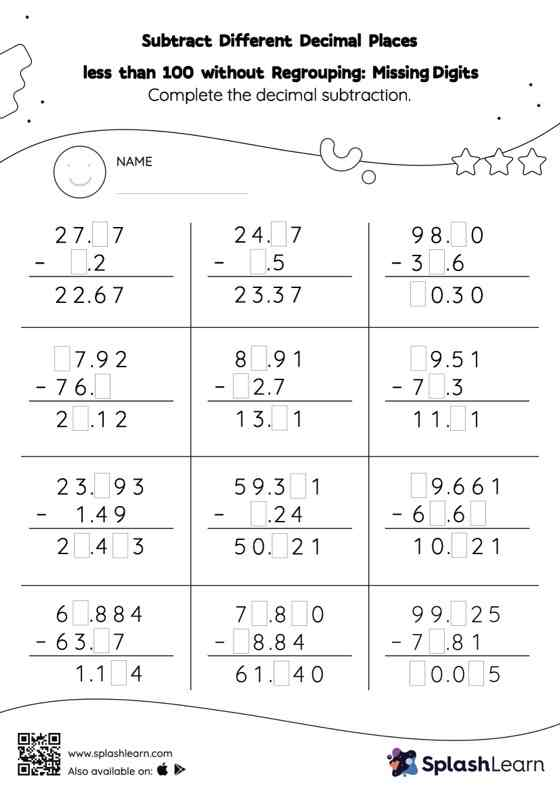# Subtract Different Decimal Places less than 100 without Regrouping: Missing Digits Worksheet

Home > Subtract Different Decimal Places less than 100 without Regrouping: Missing DigitsStudents find the missing number while subtracting decimals by using the relationship between addition and subtraction. Subtract different decimal places less than 100 without regrouping worksheet does not require them to regroup numbers. In this worksheet, students practice solving problems using the column method. This method is especially helpful with problems involving bigger multi-digit numbers as the format provides an easy structure to follow.